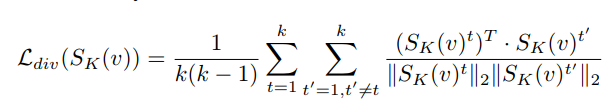# Problem about the function torch.index_select(input, dim, index, out=None)

Is this function differentiable?

Is there any implementation code behind this function? I cannot find the source code of this function in the pytorch website.

I have implemented a paper
using this function but the result is quite weird.

Other Question:
I have the problem about index differentiating below.

In this paper section 3.3

We first select Y frames (i.e. keyframes) based on the prediction scores from the
decoder.

The decoder output is [2,320], which means non-keyframe score and key frame score of the 320 frames. We want to find a 0/1 vector according to the decoder output but the process of [2,320] -> 0/1 vector seems not differentiable…

How to implement this in pytorch?

Thanks for anyone pointing out the reason.

I have found its source code.

CPU:

CUDA:

Thank you Tony-Y.
Do you think this is a differentiable function?

It is non-differentiable with respect to index.

Thank you very much, Tony-Y.

What do you think of the pytorch implementation of the “select” action in “we select k key frames to form the predicted summary video” ?

I use torch.index_select first but I know the function cannot be differentiable now.
Do you have any implementation suggestion on “select” action?

You use `index_select` 4 times in your code:

Where is the problem?

Thank you very much, Tony-Y.

The index_select function cannot be diff. so the gradient cannot backprop. to the previous S_K architecture.

My problem is how do I implement “select” action in pytorch instead of using the index_select function to implement it?

Do you want a derivative with respect to the index rather than the source tensor?

I want a derivative with respect to the source tensor[index] -> the tensor on the “index” location.

Because the output tensor of FCSN architecture is in the shape of [1,2,1,#frame]. This tensor means whether frames are selected or not.

The algo. of this paper is below:

• Downsampling every video in 2fps and pre-processing every downsampled training video to [1,1024, 1, T] (video) and [1,1024,1,S] (summary) through pre-trained googlenet.

• for every pre-processed downsample video’s feature(in the format [1,1024,1,T]) and real summary video’s feature(in the format [1,1024,1,S]): ->T,S may differ in each video

• Select K key outputs of FCSN according to index_mask and get the output in format [1,2,1,K].
• Put the selected K key outputs of FCSN([1,2,1,K]) into the 1x1 conv to get the [1,1024,1,K].
• Add K key features[1,1024,1,K] (Pick the K key features from original video feature according to index_mask) to the 1x1 conv output[1,1024,1,K] to do skip connection(this is the output of S_K).
• Pick the K key features from original video feature according to index_mask and calculate the reconstruction loss with previous step output.
• Calculate the diversity loss.
• Calculate the adv. loss by putting the output of S_K in to S_D and set target score 1 to get the adv. loss
• Update S_K.
• Put Real summary videos’ features [1,1024,1,S] into S_D to calculate and set target score 1 to get the adv. loss.
• Put Fake summary videos’ features [1,1024,1,K] come from S_K in S_D to calculate and set target score 0 to get adv. loss.
• Update S_D.
• end

Thank you very much, Tony-Y.

This reconstruction loss can be calculated by the weighted mean where the index_mask is used as the weights and k is the sum of the index_mask.

But the the frame with larger index will get more weight, am I right?

Since index_select is not used, the frame numbers of SK and v are the same.

Sorry for my poor English understanding.

Could you please set an example?

Thank you very much, Tony-Y.

``````import torch
index_mask = torch.Tensor([0.0, 0.0, 1.0, 1.0, 0.0])
v = torch.randn(3,5)
sk = torch.randn(3,5)
``````

where the feature size is 3 and the number of frames is 5.

I have followed your idea but the loss is the same quite weird.

p.s. The torch.index_select in below is just for training set preparation so I do not change.

Thank you very much, Tony-Y.

By the way, is the random selection a valid approach?

The selection is based on the output of the FCSN architecture (i.e. [1,1024,1,T] tensor)

Thank you very much, Tony-Y.

if S_K doesn’t select more than one element, then random select two element(for the sake of diversity loss)

What’s this?

Because the calculation of diversity loss must have at least two frames.I implement the diversity loss through below concept, where the feature size is 2 and the number of frames is 3.